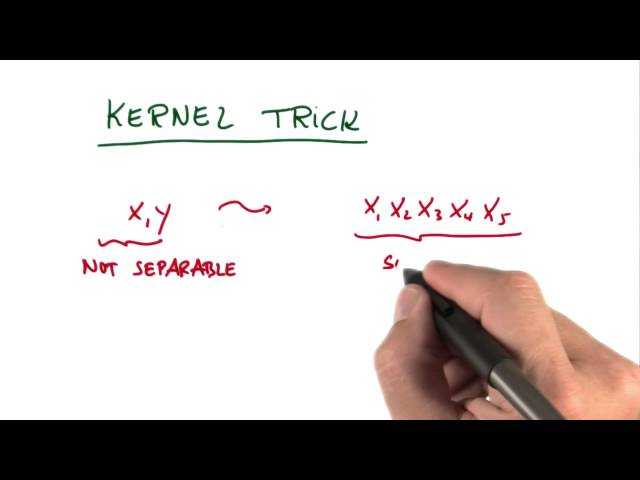# What is Kernel trick

##### The Kernel Trick in Machine Learning

The kernel trick is a technique used in machine learning to enable the use of linear algorithms for non-linear problems. It is a mathematical concept that transforms data points into a higher-dimensional space, where they can be separated by a hyperplane. The kernel trick is widely used in many applications of machine learning, including support vector machines, principal component analysis, and kernel regression.

##### Introduction to Kernels and the Kernel Trick

A kernel is a function that maps pairs of inputs, X and Y, to a number. It is used to measure the similarity between data points. Kernels are essential in machine learning because they allow algorithms to work in higher dimensions without actually computing the coordinates of those dimensions, enabling the use of linear algorithms for non-linear problems.

The kernel trick is the process of applying a kernel function to a dataset to transform it to a higher-dimensional space where a hyperplane can be used to separate the data points. The resulting separation is then projected back into the original feature space so that the classifier can use the linear algorithm. This technique avoids the computational overhead of working in a higher-dimensional space while maintaining the accuracy of non-linear algorithms.

##### Applications of the Kernel Trick

The kernel trick is widely used in many applications of machine learning, including:

• Support Vector Machines (SVMs) - SVM is a well-known algorithm for classification and regression problems. SVMs use a kernel function to transform the input data into a higher-dimensional space where a hyperplane can be used to separate the data points. The main advantage of using SVMs with the kernel trick is its flexibility to work with non-linearly separable data. The SVM achieves its optimal classification boundary by maximizing the distance between the closest data points to each class under the constraint that the data points are correctly classified.
• Principal Component Analysis (PCA) - PCA is a technique used to reduce the dimensionality of large datasets while retaining as much of the original information as possible. PCA uses the kernel trick to transform the data into a higher-dimensional space where the data can be separated using hyperplanes. This process results in a set of linearly uncorrelated principal components that explain the majority of the variation in the original data.
• Kernel Regression - Kernel regression is a technique used to estimate the values of a continuous variable based on a set of input variables. It uses the kernel trick to estimate the regression function in a high-dimensional space. The regression function is then projected back into the original feature space to make predictions.
##### Types of Kernels

There are several types of kernels that can be used with the kernel trick:

• Linear Kernel - The linear kernel is the simplest type of kernel and can be used when the data is already linearly separable. It maps the data points to the same space and computes their dot product. The resulting separation is then projected back to the original feature space.
• Polynomial Kernel - The polynomial kernel is used when the data has a non-linear relationship. It maps the data points to a higher-dimensional space using a polynomial function and computes their dot product. The degree of the polynomial determines the complexity of the function.
• Gaussian Kernel - The Gaussian kernel is the most commonly used kernel and is used when the data is non-linearly separable. It maps the data points to a higher-dimensional space using a Gaussian function and computes their dot product. The width of the Gaussian function determines the complexity of the function.
##### Advantages of the Kernel Trick

• Flexibility - The kernel trick enables linear algorithms to work with non-linearly separable data, providing greater flexibility in machine learning applications.
• Accuracy - The kernel trick is highly accurate in solving complex non-linear problems.
• Efficiency - The kernel trick avoids the computational overhead of working in a higher-dimensional space by projecting the resulting separation back into the original feature space, making it more efficient.
##### Disadvantages of the Kernel Trick

The kernel trick also has some disadvantages:

• Computational Complexity - Calculating the kernel function can be computationally expensive, especially when working with large datasets.
• Hyperparameters - The kernel function has several hyperparameters that need to be tuned to achieve optimal accuracy.
• Overfitting - The kernel trick can lead to overfitting if the kernel function is too complex or if the hyperparameters are not properly tuned.
##### Conclusion

The kernel trick is a powerful technique in machine learning that enables linear algorithms to work with non-linearly separable data. It is widely used in many applications, including support vector machines, principal component analysis, and kernel regression. The kernel trick has several advantages, including flexibility, accuracy, and efficiency, but also has some disadvantages, including computational complexity, hyperparameters, and overfitting. Properly tuning the hyperparameters is crucial to achieving optimal accuracy with the kernel trick.